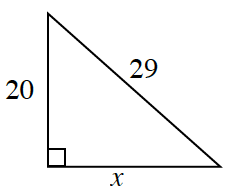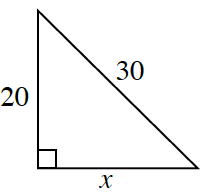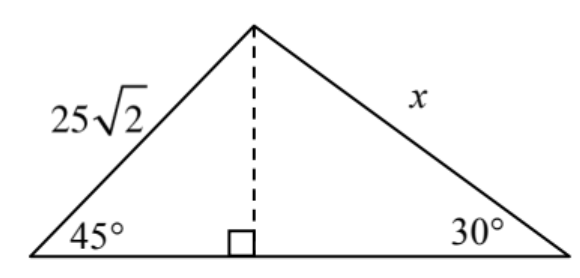### Home > CCA2 > Chapter 2 > Lesson 2.1.4 > Problem2-55

2-55.

Find the value of $x$.

1.Use the Pythagorean Theorem.

$x=21$

1.See part (a).

$x=10\sqrt{5}\approx22.3607$

1.You can use special triangles $30°-60°-90°$ and $45°-45°-90°$, or solve by using the Law of Sines.

$x=50$. Remember to check your work.